# Maharashtra Board Practice Set 14 Class 6 Maths Solutions Chapter 5 Decimal Fractions

Balbharti Maharashtra State Board Class 6 Maths Solutions covers the Std 6 Maths Chapter 5 Decimal Fractions Class 6 Practice Set 14 Answers Solutions.

## 6th Standard Maths Practice Set 14 Answers Chapter 5 Decimal Fractions

Question 1.
In the table below, write the place value of each of the digits in the number 378.025.

 Place Hundreds Tens Units Tenths Hundredths Thousandths 100 10 1 $$\frac { 1 }{ 10 }$$ $$\frac { 1 }{ 100 }$$ $$\frac { 1 }{ 1000 }$$ Digit 3 7 8 0 2 5 Place value 300 $$\frac { 0 }{ 10 }=0$$ $$\frac { 5 }{ 1000 }$$ = 0.005

Solution:

 Place Hundreds Tens Units Tenths Hundredths Thousandths 100 10 1 $$\frac { 1 }{ 10 }$$ $$\frac { 1 }{ 100 }$$ $$\frac { 1 }{ 1000 }$$ Digit 3 7 8 0 2 5 Place value 300 7 × 10 = 70 8 × 1 = 8 $$\frac { 0 }{ 10 }=0$$ $$\frac { 2 }{ 100 }$$ = 0.02 $$\frac { 5 }{ 1000 }$$ = 0.005

Question 2.
Solve :
i. 905.5 + 27.197
ii. 39 + 700.65
iii. 40 + 27.7 + 2.451
Solution:
i. 905.5 + 27.197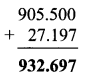ii. 39 + 700.65iii. 40 + 27.7 + 2.451Question 3.
Subtract:
i. 85.96 – 2.345
ii. 632.24 – 97.45
iii. 200.005 – 17.186
Solution:
i. 85.96 – 2.345ii. 632.24 – 97.45iii. 200.005 – 17.186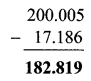Question 4.
Avinash traveled 42 km 365 m by bus, 12 km 460 in by car and walked 640 m. How many kilometers did he travel altogether? (Write your answer in decimal fractions)
Solution:
Distance traveled in bus = 42 km 365 m
= 42 km + $$\frac { 365 }{ 1000 }$$ km
= 42 km + 0.365 km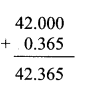= 42.365 km
Distance travelled in car = 12 km 460 m
= 12 km + $$\frac { 460 }{ 1000 }$$ km
= 12 km + 0.460 km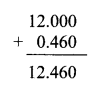= 12.460 km
Distance walked = 640 m
= $$\frac { 640 }{ 1000 }$$ = 0.640 km
∴ Total distance travelled = Distance travelled in bus + Distance travelled in car + Distance walked
= 42.365 + 12.460 + 0.640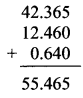= 55.465 km
∴ Distance travelled altogether by Avinash is 55.465 km.

Question 5.
Ayesha bought 1.80 m of cloth for her salwaar and 2.25 for her kurta. If the cloth costs Rs 120 per metre, how much must she pay the shopkeeper?
Solution:
Total length of cloth bought = 1.80 m + 2.25 m
= 4.05 m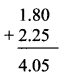Cost of 1 m of cloth = Rs 120
∴ Cost of 4.05 m of cloth = 4.05 x 120∴ Amount to be paid to the shopkeeper is Rs 486.

Question 6.
Sujata bought a watermelon weighing 4.25 kg and gave 1 kg 750 g to the children in her neighbourhood. How much of it does she have left?
Solution:
Total weight of watermelon = 4.25 kg
Weight of watermelon given to children = 1 kg 750 g
= 1 kg + $$\frac { 750 }{ 1000 }$$ kg
= 1 kg + 0.75 kg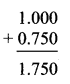= 1.75 kg
∴ Weight of watermelon left = Total weight of watermelon – Weight of watermelon given to children
= 4.25 kg – 1.75 kg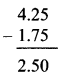= 2.5 kg
∴ Weight of watermelon left with Sujata is 2.5 kg.

Question 7.
Anita was driving at a speed of 85.6 km per hour. The road had a speed limit of 55 km per hour. By how much should she reduce her speed to be within the speed limit?
Solution:
Speed at which Anita is driving = 85.6 km per hr.
Speed limit = 55 km per hr.
∴ Anita should reduce her speed by 85.6 km per hr – 55 km per hr.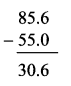= 30.6 km per hr.
∴ Anita should reduce her speed by 30.6 km per hour to be within the speed limit.

#### Maharashtra Board Class 6 Maths Chapter 4 Operations on Fractions Practice Set 14 Intext Questions and Activities

Question 1.
Nandu went to a shop to buy a pen, notebook, eraser and a paint box. The shopkeeper told him the prices. A pen costs four and a half rupees, an eraser one and a half, a notebook six and a half and a paintbox twenty-five rupees and fifty paise. Nandu bought one of each article. Prepare his bill.
If Nandu gave a 100 rupee note, how much money does he get back? (Textbook pg. no. 29)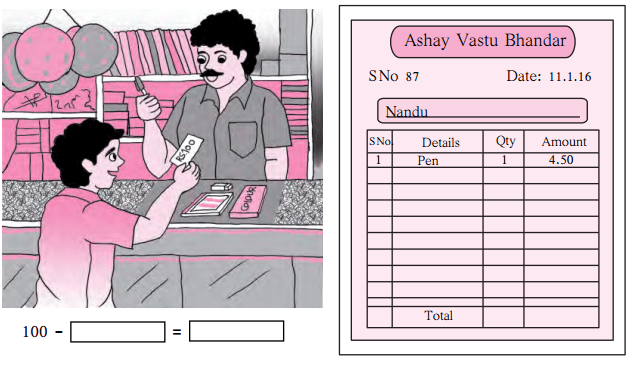Nandu will get __ rupees back.
Solution:
100 – 38 = 62.00
Nandu will get Rs 62 rupees back.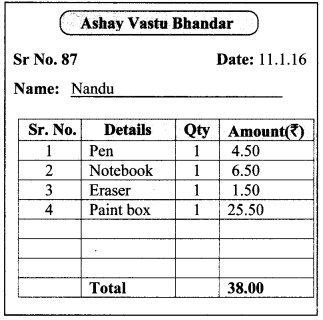Question 2.
Take a pen and notebook with you when you go to the market with your parent. Note the weight of every vegetable your mother buys. Find out the total weight of those vegetables. (Textbook pg. no. 30)
Solution:
(Students should attempt this activity on their own.)

Scroll to Top